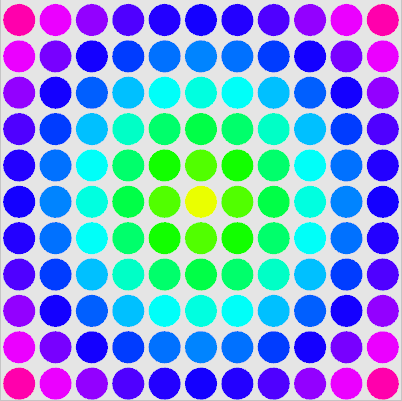## sketch experiment 6 - color

For my next sketch experiment I played with the hsb command to create animated colorsWhat is a litte bit wired in sketch is how the sketch width and height affect the window size - i originally planned to resize the window to fit 11 circles each 40 pixel in diameter - but the only windowsize where the drawing area is centered seems to be 400 - not yet sure why - i will try to dig into the sourcecode of the sketch macro and figure out what happens here

here is the source code of my experiment

``````(defsketch blub (
(title "sketch ex6")
(width 400)
(height 400)

(f 0)
(p 0)
(w (/ 400 11))
)
(incf f 0.01)
(background (rgb .9 .9 .9))

(dotimes (i 11)
(dotimes (j 11 )
(setf dx (- 5 i))
(setf dy (- 5 j))
(if (> f 1) (setf f (- f 1)))

(setf p (+ f (* .1 (sqrt (+ (* dx dx) (* dy dy))))))
(if (> p 1) (setf p (ffloor p)))

(push-matrix)
(translate (+ (/ w 2) (* i w)) (+ 20 (* j w)))
(with-pen (make-pen :fill (hsb p 1 1))
(ellipse 0 0 (- (/ w 2) 2) (- (/ w 2) 2) ))
(pop-matrix)
)
)
)
``````